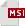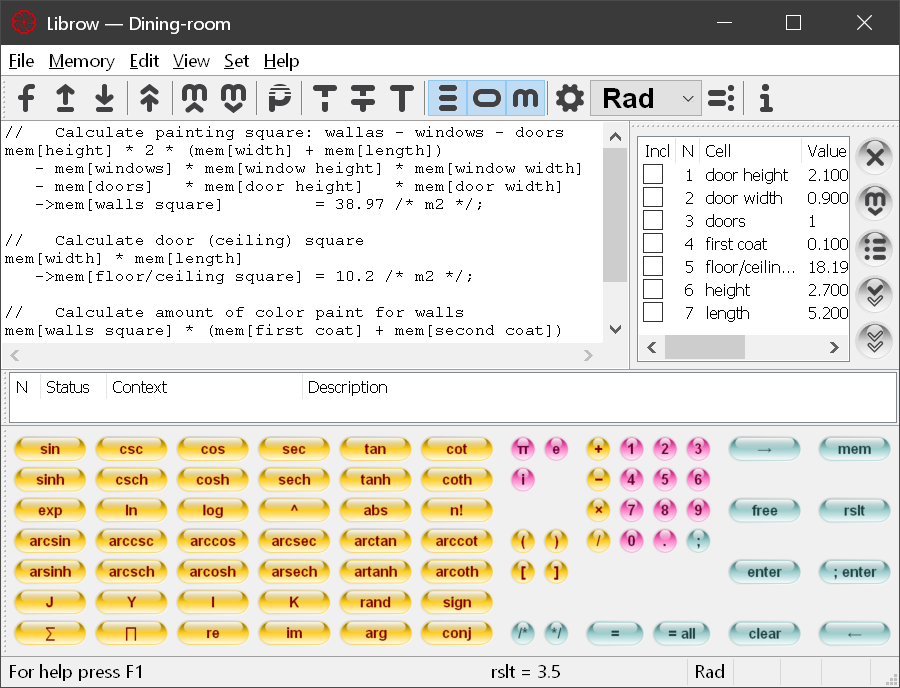The Art of Mathematics

# Professional Formula Calculator

## Professional version

 For science and engineering — complex numbers and extended function setDownload link is coming soon (msi, 8.1 MB)Fig. 1. Librow professional formula calculator.

## Complex numbers

 All functions support complex argument

Librow calculator accepts both i and j as imaginary unit:

``````sin(1+i*2)
sin(1+j*2)``````

The calculator natively supports real and imaginary parts, argument and conjugate of the complex number.

Real and imaginary parts of the number z stored in memory:

``````re(mem[z])
im(mem[z])``````
``````arg(rslt)
conj(rslt)``````

## Bessel functions

 Both ordinary and modified Bessel functions of fractional order and complex argument

Librow calculator supports four Bessel functions of fractional order.

``J(1.5, 1+i*2)``
``Y(1.5, 1+i*2)``
``I(1.5, 1+i*2)``
``K(1.5, 1+i*2)``

## Gamma function

 Gamma function — extension of factorial to complex numbers

Librow calculator supports gamma function:

``Γ(1+i*2)``

or:

``Gamma(1+i*2)``

## Sum and product of series

 Native functions for sum and product of series

Librow calculator can easily calculate sum and product of long series:

``````//   Sum of 1/k series, k runs from 1 to 100
∑(k, 1, 100, 1/k)

//   Product of 1/k series, k runs from −1 to −100
∏(k, −1, −100, 1/k)``````

Support of arbitrary index step:

``````//   Sum of 1/(k+1) series, k runs from 0 to 100 with a step of 4
∑(k, 0, 100, 4, 1/(k+1))``````

## Random numbers

 Embedded random number generator

The calculator generates random numbers in [0, 1] range:

``arcsin(rand)``

## Ceiling and floor functions

 Transforms of reals to integers

Librow calculator supports both ceiling and floor functions:

``````ceil(−1.2)
floor(1.4)``````

## Technical data

 Functional, robust, professional

Precision: double

Number input and output format: decimal (standard notation) and exponential (scientific notation)

Supported operators: addition, subtraction, multiplication, division

Supported functions:

RAM: min 4 MB

Size on disk: application — 3.9 MB, its help — 2.1 MB

Platform: Windows XP, Windows Vista, Windows 7, Windows 8, Windows 10; 32-bit and 64-bit

## Installation

 msi — Microsoft Installation for professional administrationDownload link is coming soon (msi, 8.1 MB)